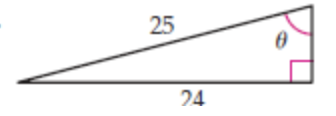##### Find the equation of the plane through the point (1,1,1) which is perpendicular to the line of intersection of the two planes x−y−3z=−1 and x−3y+z= 2.

Find the equation of the plane through the point (1,1,1) which is perpendicular to the line of intersection of the two planes x−y−3z=−1  and x−3y+z= 2.

##### Solve the 4 degree polynomial equation.

Solve the 4 degree polynomial equation $$x^4 − 2x^3 + 4x^2+ 6x − 21 = 0$$, given that the sum of two its roots is zero.

##### Find the values of sin θ, cos θ, and tan θ for the given right triangle. Give the exact values.

Find the values of sin θ, cos θ, and tan θ for the given right triangle. Give the exact values.##### Which of the following sets are not well defined? Explain.

Which of the following sets are not well defined? Explain.

a. The set of wealthy school teachers

b. The set of great books

c. The set of natural numbers greater than 100

d. The set of subsets of $$\{1,2,3,4,5,6\}$$

e. The set $$\{x \mid x \neq x$$ and $$x \in N\}$$